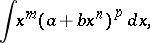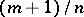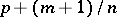# Differential binomial

(diff) ← Older revision | Latest revision (diff) | Newer revision → (diff)
Jump to: navigation, search

An expression of the typewhereandare real numbers, while,andare rational numbers. The indefinite integral of a differential binomial,is reduced to an integral of rational functions if at least one of the numbers,andis an integer. In all other cases, the integral of a differential binomial cannot be expressed by elementary functions (P.L. Chebyshev, 1853).

#### Comments

The statement on the reduction to an integral of rational functions is called the Chebyshev theorem on the integration of binomial differentials.

How to Cite This Entry:
Differential binomial. Encyclopedia of Mathematics. URL: http://www.encyclopediaofmath.org/index.php?title=Differential_binomial&oldid=11396
This article was adapted from an original article by L.D. Kudryavtsev (originator), which appeared in Encyclopedia of Mathematics - ISBN 1402006098. See original article